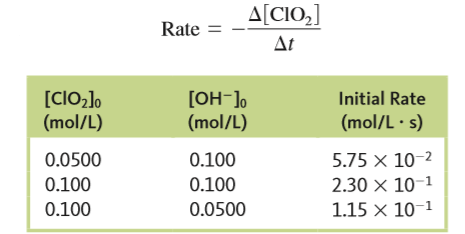# Problem: The following data were obtained for the reaction2ClO2 (aq) + 2OH- (aq) → ClO3- (aq) + ClO2- (aq) + H2O (l)wherea. Determine the rate law and the value of the rate constant.

###### Problem Details

The following data were obtained for the reaction

2ClO(aq) + 2OH(aq) → ClO3- (aq) + ClO2- (aq) + H2O (l)

wherea. Determine the rate law and the value of the rate constant.